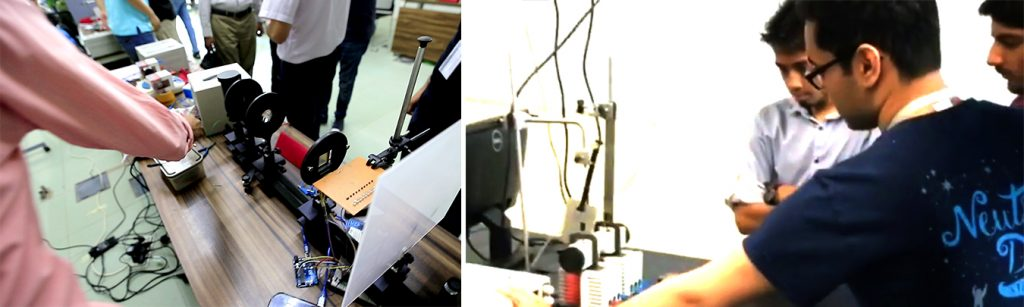Habib Response - Covid-19# Phy101L: Mechanics Lab

Phy101L course compliments the Phy101 course in Mechanics. The lab is based on several theoretical concepts that the students learn during the course. The lab has the following experimental setups:

Uncertainties in measurements
This is the most basic experiment where students learn how to compute type A and B uncertainties, how to combine and transfer from one variable to another. The experiment we use to conduct this exercise it to compute value of gravitational acceleration using a simple pendulum with different length of pendulums.
Expansion of Helical spring
We observe the extension of springs in series and parallel combinations and compare it with mathematical models that we derive using Hooke’s Law.
Damping Constant of a Harmonic Oscillator
A pendulum made up of a neodymium magnet is made to oscillate between two sets of coils with 250 turns. The oscillating magnet causes magnetic flux that induces voltage in the coil. As the pendulum oscillates its amplitude decreases with time by a certain factor, namely Damping Constant. Readings of voltage is recorded and using the method of curve fitting, value of damping constant is determined.
Energy Conservation in 2-Dimension
A ball at a certain height is hit in horizontal by a pendulum that has been released at different angles. The ball then moves horizontally because of the collision and also vertically downwards due to force of gravity until it touches ground at certain distance. Using the concepts of projectile motion and energy conservation, it can be mathematically modelled and can be tested against experimental data.
Conservation of Linear Momentum
Collisions of two trolleys are being observed for elastic and inelastic with equal and not equal masses to determine if the kinetic energy and liner momentum of the system is conserved or not and why.
Rotational Motion and frictional losses
The experiment aims to build understating the motion and transfer of energy of an oscillating mass. It also focuses on relationship of inertia and frictional losses.
Path diagram of rotational motion
We just directly replace the variables from the equation of kinematics of linear motion with variables of rotational motion, and observe if the concepts would still complement the experimental data. In the experiment we measure angular displacements, time to travel from one angle to another and instantaneous angular velocities.
Energy Conservation in Maxwell’s Wheel
A wheel with a perpendicular spindle at its centre of known moment of inertia is wounded up with thread and then released to oscillate vertically due to gravity. As it unwinds, the wheel has translational motion and as well as rotational motion. By recording the instantaneous translational velocity at different heights from drop, we compute experimental value of moment of inertia. We conclude that energy remains conserved at all times.
Latent Heat of Vaporization of Liquid Nitrogen
Liquid Nitrogen boils at -196 degree Celsius. We measure the rate of mass loss of liquid nitrogen due environmental heating at room temperature and then by providing external electrical heating. By computing the difference in rate and power of electrical heating, using the formula of Latent heat of vaporization, we determine the experimental value, and then compare it with literature value.
Newton’s Law of Cooling
We determine the coefficient of convective heat transfer between a hot metallic cylinder as it cools down and surrounding air. We also determine how the coefficient of convective heat transfer changes in different conditions.
Determination of Curie temperature of Kanthal D-wire
Curie temperature of a material is the temperature at which a material losses its permanent magnetic field. In this experiment we heat a spring made of Kanthal D-wire by electric heating up to a point where it no longer responds to external magnetic field. The curie temperature is then determined for a range of voltages to get an accurate enough value.
Vibrations on a String and Resonance
In this experiment standing waves are induced and observed on a steel string. We identify at what frequency resonance occur in the given strings, and compare experimental data with mathematical relationships.